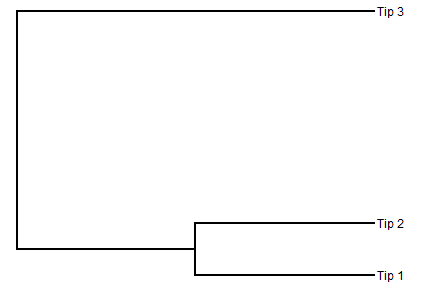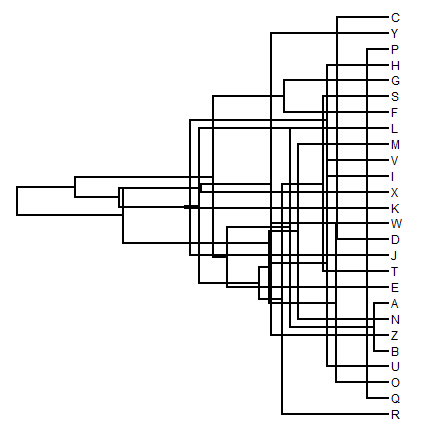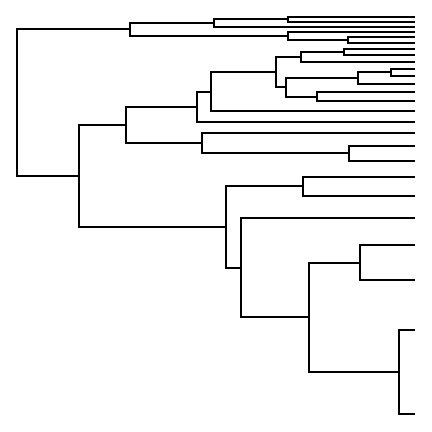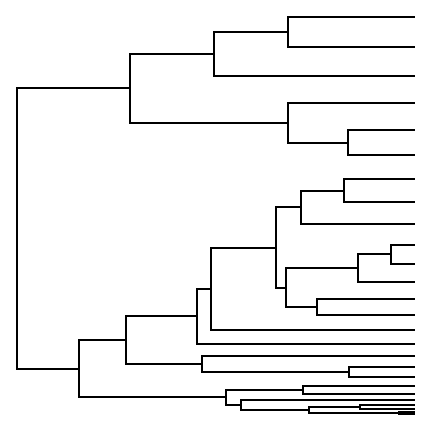## Friday, January 30, 2015

### Arbitrary vertical spacing of the tips on a plotted tree

I am looking for a way to manually specify varying vertical distance between the tips in a phylogeny (because i want to add several lines of text to some of the tips).

I.e. for the tree library(ape) TREE=read.tree(text=“((Tip_1:1,Tip_2:1):1,Tip_3:2);)”)

Is there a way for instance through plot phylo to print place the tips in the heights of c(1,2,6) as opposed to c(1,2,3) as they would be normally in plot.phylo.

This is not presently possible, but I realized immediately that this would be pretty straightforward to add to phytools `plotSimmap` because the way that functions works is by first assigning `1:N` to the `N` tips of the tree, and then works backwards to assign the vertical position of all internal nodes via a post-order traversal.

I responded to the query, and now I have also posted code online that permits exactly this type of manipulation. The new phytools build with this update can be obtained here. Here's a demo using the code of the original query:

``````library(ape)
library(phytools)
packageVersion("phytools")
``````
``````##  '0.4.43'
``````
``````## normal vertical spacing
plotTree(TREE)
````````````## modified spacing
tips<-setNames(c(1,2,6),TREE\$tip.label)
tips
``````
``````## Tip_1 Tip_2 Tip_3
##     1     2     6
``````
``````plotTree(TREE,tips=tips)
``````Note that there is nothing here to prevent us from plotting trees with line crossing! For instance:

``````tree<-pbtree(n=26,tip.label=LETTERS)
tips<-setNames(1:26,sample(LETTERS))
plotTree(tree,tips=tips)
``````Yikes!

We can also do other weird stuff, like this:

``````plotTree(tree,tips=setNames(log(1:26),tree\$tip.label),ftype="off")
````````````plotTree(tree,tips=setNames((1:26)^2,tree\$tip.label),ftype="off")
``````Well, you get the idea.

That's it.

#### 1 comment:

1.As a student and reader of R-syg-phylo, same question also arose in my mind that how to best essays reviews specify the vertical distance between the tips of polygene manually. Your answer has satisfied my hunger and it surely it will satisfy others.

Note: due to the very large amount of spam, all comments are now automatically submitted for moderation.